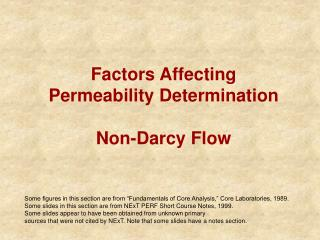DownloadDownload PresentationFactors Affecting Permeability Determination Non-Darcy Flow

# Factors Affecting Permeability Determination Non-Darcy Flow

Download Presentation## Factors Affecting Permeability Determination Non-Darcy Flow

- - - - - - - - - - - - - - - - - - - - - - - - - - - E N D - - - - - - - - - - - - - - - - - - - - - - - - - - -
##### Presentation Transcript

1. Factors Affecting Permeability Determination Non-Darcy Flow Some figures in this section are from “Fundamentals of Core Analysis,” Core Laboratories, 1989. Some slides in this section are from NExT PERF Short Course Notes, 1999. Some slides appear to have been obtained from unknown primary sources that were not cited by NExT. Note that some slides have a notes section.

2. Air Permeability Measurement • Measurement of permeability in the laboratory is most commonly done with air • Convenient and inexpensive • Problem: low values of mean flowing pressure • downstream pressure, patm • upstream pressure, just a few psi higher than patm • At low mean flowing pressure, gas slippage occurs • Diameter of flow path through porous media approaches the “mean free path” of gas molecules • mean free path is a function of molecule size • mean free path is a function of gas density • Increasing mean flowing pressure results in less slippage • as pmean, we obtain absolute (equivalent liquid) permeability

3. Non-Darcy Flow - Gas Slippage • Liquid flow and gas flow at high mean flowing pressure is laminar • Darcy’s Law is valid • flow velocity at walls is zero • At low mean flowing pressure gas slippage occurs • Non-Darcy flow is observed • flow at walls is not zero • Klinkenberg developed a method to correct gas permeability measured at low mean flowing pressure to equivalent liquid permeability

4. Non-Darcy Flow - Klinkenberg Effect • As pmean, gas permeability approaches absolute permeability

5. Non-Darcy Flow - Klinkenberg Effect • Klinkenberg correction for kair depends on mean flowing pressure • correction ratio shown is for pmean = 1 atm

6. Non-Darcy Flow - Klinkenberg Effect • Klinkenberg correction for kair is more important for low absolute permeability

7. Non-Darcy Flow - High Flow Rates • In the field, gas wells exhibit non-Darcy flow at high flow rates • At high flow velocity, inertial effects and turbulence become important, and cause non-Darcy flow • inertial effect

8. Non-Darcy Flow - Turbulence • Recalling Darcy’s equation for gas flow, (zg )=Constant • For laboratory flow experiments we can assume T=Tsc and z=1 • For Darcy flow, plotting (qg,sc psc)/A vs. (p12-p22)/(2L) results in straight line. • line passes through origin [when qg,sc =0, then (p12-p22)=0] • slope = k/ g • behavior departs from straight line under turbulent flow conditions (high flow velocity)

9. Darcy flow Non-Darcy flow q psc A k Slope = m 0 0 (p12- p22) 2 L Non-Darcy Flow - Turbulence

10. Non-Darcy Flow - Forchheimer Equation • Forchheimer proposed a flow equation to account for the non-linear effect of turbulence by adding a second order term • Note that unit corrections factors would be required for non-coherent unit systems. • As flow rate decreases, we approach Darcy’s Law (2nd order term approaches zero)

11. Non-Darcy Flow - Forchheimer Plot • Based on Forchheimer’s Equation a plotting method was developed to determine absolute permeability even with Non-Darcy effects • (1/kgas) vs. qg,sc • kgas determined from Darcy’s Law (incorrectly assuming Darcy flow) and is a function of qg,sc • intercept = (1/kabs); absolute permeability (1/kgas), (1/md) Slope = [(brg,sc)/(mgA)] Intercept = [1/kabs] qg,sc

12. Non-Darcy Flow - Forchheimer Equation • Non-Darcy Coefficient, b, is an empirically determined function of absolute permeability • For Travis Peak (Texas) NOB=Net Overburden